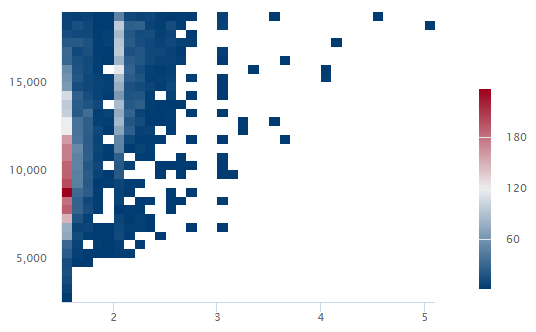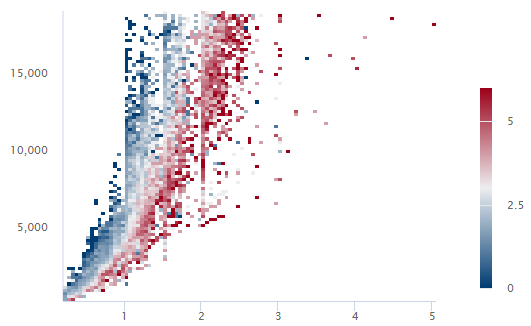# Enter your data for Binned Scatterplot

Graph > Binned Scatterplot

Enter the x- and y-variables, define the gradient, select the scales to make similar, and enter the by variables.

Graph > Binned Scatterplot

## Enter the Y-variable and X-variable

Graph > Binned Scatterplot

You can graph the x and y-variables as individual pairs or you can graph every combination of the x-y variables. The y-variable is the variable that you want to explain or predict. The x-variable is a corresponding variable that might explain or predict changes in the y-variable. All columns must be numeric, and each x-y variable pair must have the same number of rows.

### X-Y pairs

Select to display a separate graph for each pair of x and y-variables that you enter. In this worksheet, Debt Ratio is the y-variable and Age is the x-variable. The graph shows the relationship between debt ratio and age.

### Each Y versus each X

Select to display a separate graph for each possible combination of x and y-variables that you enter. In this worksheet, Debt Ratio is the y-variable and Age and Income are the x-variables. Minitab creates two graphs, one that shows the relationship between debt ratio and age and another that shows the relationship between income and age.

## Select what the gradient is defined by

Graph > Binned Scatterplot

Select how you want to define the color scale of the bins on the binned scatterplot.

Frequency of binned data
Select to define the gradient scale by the number of observations in the bin.In this example, bins with 180 observations or more are dark red. Bins with 60 observations or fewer are dark blue. Bins with between 60 and 180 observations are lighter shades of blue, red, and gray.
Mean of variable
Select to define the gradient scale by the value of a third variable.In this example, when the average value of all observations of the third variable is greater than 5, the bin is dark red. When the average value of all observations of the third variable is less than 1, the bin is dark blue. Bins with average values between 1 and 5 are lighter shades of blue, red, and gray.

## Select the scales to make similar

Graph > Binned Scatterplot
Use the same scale across multiple graphs. These options become available when you enter more than one pair of columns in X-variable and Y-variable.
Same X-scale
Use the same x-scale across all graphs.
Same Y-scale
Use the same y-scale across all graphs.

## Enter the By variables

Graph > Binned Scatterplot
Enter one or more grouping variables in By variables to create a separate binned scatterplot for each level of the grouping variables. The columns that you enter can be numeric or text, and must be the same length as the columns in X-variable and Y-variable. The y-scales for each variable are the same across the multiple binned scatterplots.
Show all combinations

When you enter multiple By variables, Minitab enables the Show all combinations checkbox. Select this option to create a separate binned scatterplot for each combination of groups created by the By variables. If you do not select this option, Minitab creates a plot for each group of each By variable.

For example, the first By variable has 2 groups, Male and Female, and the second By variable has 2 groups, Employed and Unemployed. If you select Show all combinations, Minitab creates 4 separate plots for the combinations of Male/Employed, Male/Unemployed, Female/Employed, and Female/Unemployed. If you do not select Show all combinations, Minitab creates 4 separate plots for Male, Female, Employed, and Unemployed.

By using this site you agree to the use of cookies for analytics and personalized content.  Read our policy